Home > MC2 > Chapter 11 > Lesson 11.1.1 > Problem11-10

11-10.
1. Use the laws of exponents to simplify the following expressions. Homework Help ✎

1. (x2)(x5)

2.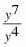3. x3 · x4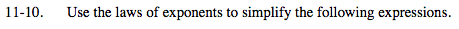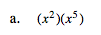When multiplying two terms with the same base, add the exponents together.

x(2 + 5)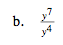When dividing two terms with the same base, subtract the exponent in the denominator from the exponent in the numerator.

y(7 − 4)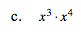See part (a).